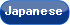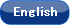Please use this identifier to cite or link to this item: `http://hdl.handle.net/10119/4919`

 Title: Pseudo approximation algorithms with applications to optimal motion planning Authors: Asano, TetsuoKirkpatrick, DavidYap, Chee Issue Date: 2004-01 Publisher: Springer Magazine name: Discrete and Computational Geometry Volume: 31 Number: 1 Start page: 139 End page: 171 DOI: 10.1007/s00454-003-2952-3 Abstract: We introduce a technique for computing approximate solutions to optimization problems. If X is the set of feasible solutions, the standard goal of approximation algorithms is to compute x ∈ X that is an ε - approximate solution in the following sense: d(x) ≤ - (1+ε)d(x^*), where x^* ∈ X is an optimal solution, d : X → R ≥ 0 is the optimization function to be minimized, andε>0 is an input parameter. Our approach is first to devise algorithms that compute pseudo ε-approximate solutions satisfying the bound d(x) ≤ - d(x^*_R) +εR, where R>0 is a new input parameter. Here x^*_R denotes an optimal solution in the space X_R of R-constrained feasible solutions. The parameter R provides a stratification of X in the sense that (1) X_R ⊆ X_ for R < R’ and (2) X_R = X for R sufficiently large. We first describe a highly efficient scheme for converting a pseudo ε-approximation algorithm into a trueε-approximation algorithm. This scheme is useful because pseudo approximation algorithms seem to be easier to construct than ε-approximation algorithms. Another benefit is that our algorithm is automatically precision-sensitive. We apply our technique to two problems in robotics: (A) Euclidean Shortest Path (3ESP), namely the shortest path for a point robot amidst polyhedral obstacles in 3D, and (B) d_1-optimal motion for a rod moving amidst planar obstacles (1ORM). Previously, no polynomial time ε-approximation algorithm for (B) was known. For (A), our new solution is simpler than previous solutions and has an exponentially smaller complexity in terms of the input precision. Rights: This is the author-created version of Springer, Tetsuo Asano, David Kirkpatrick and Chee Yap, Discrete and Computational Geometry, 31(1), 2004, 139-171. The original publication is available at www.springerlink.com, http://dx.doi.org/10.1007/s00454-003-2952-3 URI: http://hdl.handle.net/10119/4919 Material Type: author Appears in Collections: b10-1. 雑誌掲載論文 (Journal Articles)

Files in This Item:

File Description SizeFormat